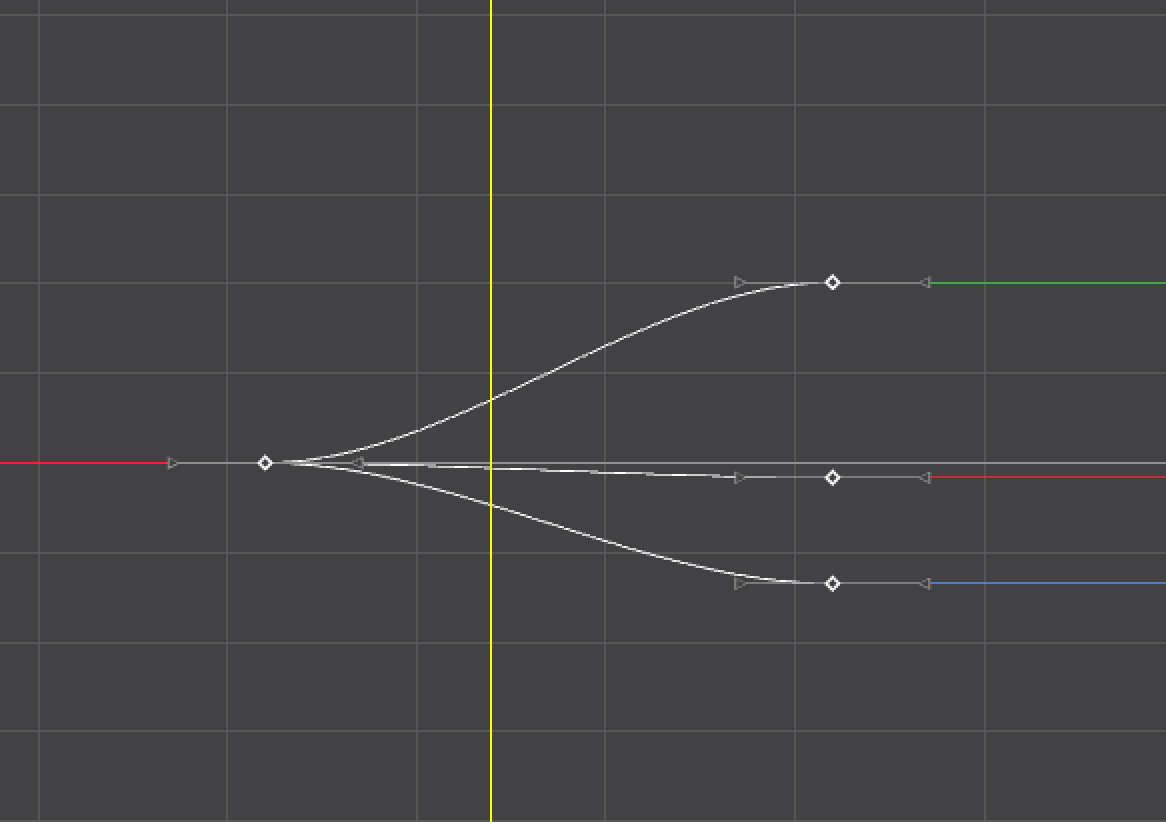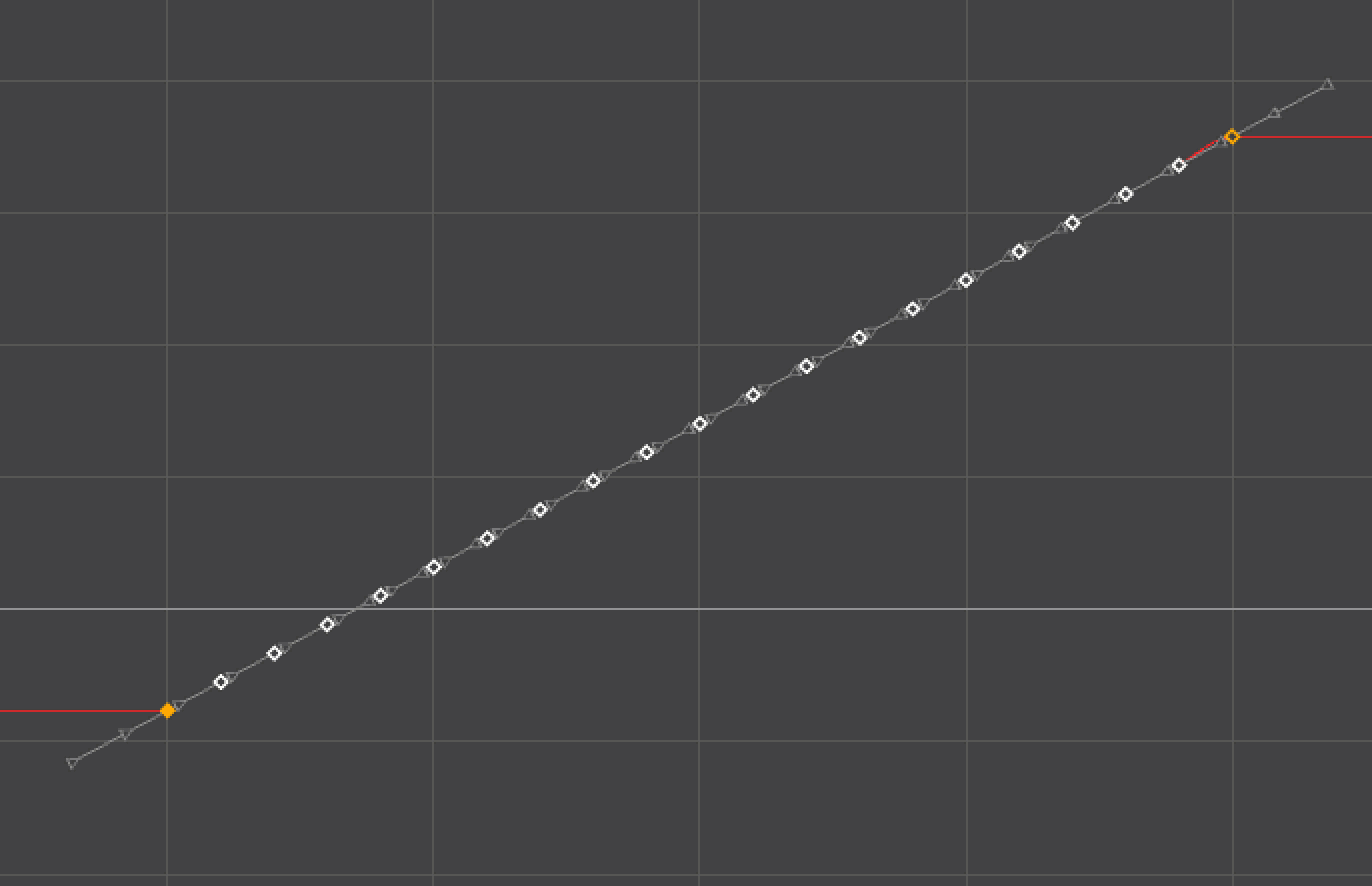# Synopsis

 setSlider(slider=str, value=float, frame=float)

This command is equivalent to use an animBot slider. It acts on selected keys in the Graph Editor, otherwise on range selected on the Timeline or current frame.

# Flags

 Name Argument types Description slider enum Sets the slider to be used. Available sliders: kBlendToBufferkBlendToDefaultkBlendToEasekBlendToFramekBlendToFrameWorldSpacekBlendToInfinitykBlendToInfinityWorldSpacekBlendToMirrorkBlendToNeighborskBlendToNeighborsWorldSpacekBlendToUndokConnectToNeighborskEaseInOutkGapStitcherkMirrorToFramekNoiseWavekPullPushkScaleFromAveragekScaleFromDefaultkScaleFromFramekScaleFromNeighborLeftkScaleFromNeighborRightkSimplifyBakeKeyskSmoothRoughkTimeOffsetterkTimeOffsetterStaggerkTweenerkTweenerWorldSpace value float Sets the value to be applied, -100 is equivalent to use the slider all the way to the left, 100 is equivalent to all the way to the right. Greater values are equivalent to overshoots. frame float Optional. Sets the frame for sliders with frame pick, such as kBlendToFrame.

# Examples

This is equivalent to use Tweener 1/3:

```from animBot import OpenAnimBot as OA

OA.setSlider(OA.kTweener, -33)

```

This will bake keyframes and make a noise within the range selected in the Graph Editor:```from animBot import OpenAnimBot as OA

OA.setSlider(OA.kSimplifyBakeKeys, 100)
OA.setSlider(OA.kNoiseWave, -100)```

This will blend to frame 27:

```from animBot import OpenAnimBot as OA

OA.setSlider(OA.kBlendToFrame, 100, frame=27)```

Making something a little more complex, the command below will blend to ease in and out at the same time:```from maya import cmds
from animBot import OpenAnimBot as OA

# play with those two values
EASING_AMOUNT = 5
MIDDLE_POINT = 30 # 0 to 100

animCurvesMap = {}
animCurves = cmds.keyframe(query=True, name=True, selected=True) or []

for animCurve in animCurves:

keyTimes = cmds.keyframe(animCurve, selected=True, query=True, timeChange=True) or []

animCurvesMap[animCurve] = keyTimes

cmds.undoInfo(openChunk=True)

try:

for animCurve, keyTimes in animCurvesMap.items():

middlePoint = int(len(keyTimes) / (100. / MIDDLE_POINT))

# select first half
firstHalf = keyTimes[:middlePoint]
cmds.selectKey(animCurve, time=(firstHalf, firstHalf[-1]))

OA.setSlider(OA.kBlendToEase, -EASING_AMOUNT)

# select second half
secondHalf = keyTimes[middlePoint:]
cmds.selectKey(animCurve, time=(secondHalf, secondHalf[-1]))

OA.setSlider(OA.kBlendToEase, EASING_AMOUNT)

# reselect keys
for animCurve, keyTimes in animCurvesMap.items():